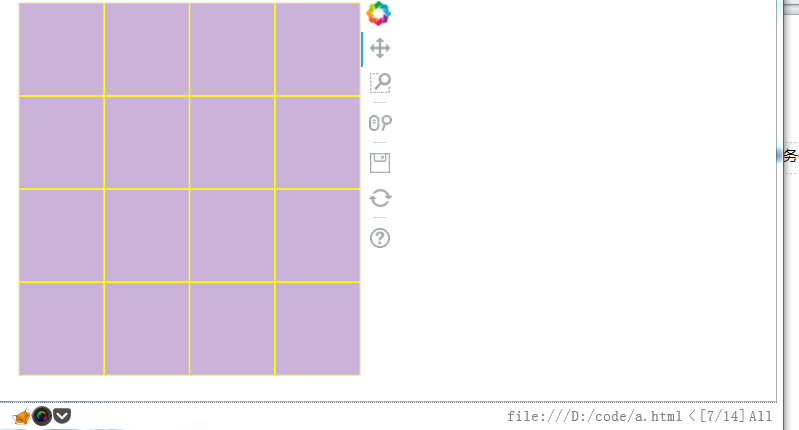# How can I split full screen evenly with scale_both?

Hi, all
What I am trying to do is to make a figure, which is the size of the current screen and then split evenly into smaller rectangles.

in the code below, I managed to split a figure into 9 rects, but the problem is that the figure does not scale according to the width, it fits the height only
from bokeh.layouts import row
import math
from bokeh.plotting import figure, show, output_file

number = 15

size = int(math.sqrt(number)) + 1
output_file(“a.html”)

x =
y =
for i in range(size):
# get x
for j in range(size):
y.append(i+0.5)
x.append(j + 0.5)

print(x, y)
p = figure(x_range=[0, 4], y_range=[0, 4])

p.sizing_mode = ‘scale_both’
p.xaxis.visible = False
p.yaxis.visible = False

r = p.rect(x, y, width=1, height=1, color="#CAB2d6",)
glyph = r.glyph
glyph.line_color = ‘yellow’
show§

``

screenshot below,it is possible to scale the figure to the full browser and then split them. I would assume the code x_range, y_range should be updated to something else?